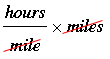SEARCH HOMEMath Central Quandaries & QueriesQuestion from Willie, a parent: If you travel at 50 mph, how long does it take to go 45 miles?Hi Willie,

I know it's a little less than 1 hour since in 1 hour you would go 50 miles.

The units tell you what to do. You have two quantities, one in miles/hours and the other in miles and you want an answer in hours. Thus I wantThus my calculation is

1/50 × 45 = 5/50 = 0.9 hours.

If you want that in minutes then, since there are 60 minutes in an hour

0.9 hours = 0.9 × 60 = 54 minutes.

I hope this helps,
PennyMath Central is supported by the University of Regina and The Pacific Institute for the Mathematical Sciences.